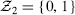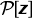## 8.3 THE ALGEBRA OF POLYNOMIALS OVERwill denote the set of polynomials in the variable z whose coefficients {pi} are in:Arithmetic operations on polynomials are the usual, except that the addition and multiplication of coefficients is performed modulo 2. We write deg(p) for the degree of pThe subset ofconsisting of polynomials with deg(p) ≤ n will be denoted by Pn[z]. We next summarize several basic properties of.

### 8.3.1 Properties of1. fhas a factorization, if f(z) = g(z)h(z) with g, h . If f(z) = g(z)h(z), then g(z) and h(z) are factors of f(z)

(a)    f(z) = g(z)h(z) is a non-trivial ...

Get Computer Security and Cryptography now with the O’Reilly learning platform.

O’Reilly members experience live online training, plus books, videos, and digital content from nearly 200 publishers.